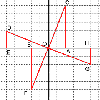#### You may also like### 8 Methods for Three by One

This problem in geometry has been solved in no less than EIGHT ways by a pair of students. How would you solve it? How many of their solutions can you follow? How are they the same or different? Which do you like best?### Reflect Again

Follow hints to investigate the matrix which gives a reflection of the plane in the line y=tanx. Show that the combination of two reflections in intersecting lines is a rotation.### The Matrix

Investigate the transfomations of the plane given by the 2 by 2 matrices with entries taking all combinations of values 0. -1 and +1.

# Rots and Refs

##### Age 16 to 18Challenge Level

Bob sent us his solution:

Firstly I multiplied the matricies to find the new point. Using the trigonometric identities to simplify, I got the new point as $(r\cos(\theta+\phi),r\sin(\theta+\phi))$. This meant that we had rotated the point $\phi$ degrees anticlockwise.

To prove that $OX = OX' = p$, I drew a line XX', which intersects and is parallel to the line $y=x\tan\theta$ (call this point of intersection D). But $DX'=DX$ and so $ODX$ and $ODX'$ are two right-angled triangles of the same size, so $OX=OX'=p$. By the same argument I drew lines $OP$ and $OP'$, and so got right-angled triangles again, so $OP=OP'=q$.

By looking at the right-angled triangle $OAX'$, with the angle at $O$ being $2\theta$, I knew that: $$\cos2\theta=\frac{OA'}{OX}=\frac{OA'}{p}$$ and so $OA'=p\cos2\theta$.

I then looked at the right-angled triangle $X'BP$, and since the angle at $X$ is $2\theta$, $BP'=q\sin2\theta$. By applying Pythagoras' Theorem to the right-angled triangle $OAX$,$AX'=p\sin2\theta$. Finally, applying Pythagoras' Thereom to the triangle $X'BP'$ I found $BX'=q\cos2\theta$.

Looking at the change in X co-ordinates, I found $P'=(p\cos2\theta+q\sin2\theta, \; p\sin2\theta-q\cos2\theta)$.

So the matrix for the reflection would be:
\mathbf{T}= \left( \begin{array}{cc} cos 2\theta & sin2\theta\\ sin2\theta & -\cos2\theta \end{array} \right)

You may also like to look at the problem ' The Matrix ' from July 2003 and its solution for an explanation of how a transformation of the plane is given by a matrix and how you can find the image of a point by multiplying its vector by the matrix of the transformation.